# Topological Sort

One of the things I generally say about myself is that I love learning. I can spend hours upon hours reading papers and algorithms to better understand a topic. Some of these topics are stand alone segments that I can understand in one sitting. Sometimes, however, there is a need to read up on some preliminary work in order to fully understand a concept.

Lets say that I was interested in organizing this information into a new course. The order I present these topics is very important. Knowing which topics depend on one another allows me to use the topological sorting algorithm to determine an ordering for the topics that respects the preliminary work.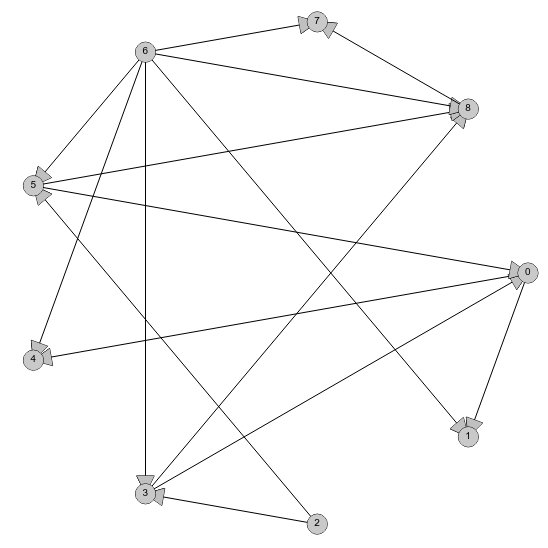The input for the topoligical sorting algorithm is a Directed Acyclic Graph (DAG). This is a set of relationships between pairs of topics, where if topic 1 must be understood before topic 2, we would add the relationship (topic 1, topic 2) to the graph. DAGs can be visualized by a set of nodes (points) representing the topics. Relationships like the one above (topic 1, topic 2) can then represented by a directed arc originating at topic 1 and flowing in the direction of topic 2. We say that the graph is “Acyclic” because there cannot be a cycle in the topic preliminaries. This amounts to us saying that a topic cannot be a prerequisite for itself. An example of a DAG is shown in the image above.

With the topics represented as a DAG, the topologial ordering algorithm works by searching the set of nodes for the one with no arcs coming into it. This node (or these nodes is multiple are present) represents the topic that can be covered next without losing understanding of the material. Such a node is guaranteed to exist by the acyclic property of the DAG. Once the node is selected, we can remove this node as well as all arcs that originate at this node from the DAG. The algorithm then repeats the procedure of searching for a nod with no arcs coming into it. This process repeats until there are no remaining nodes from which to choose.

Now lets see how the topological sort algorithm works on the graph above. We will first need to count the in-degree (the number of arcs coming into) each node.

Node | Indegree
—————-
0 | 2
1 | 2
2 | 0
3 | 2
4 | 2
5 | 2
6 | 0
7 | 2
8 | 3

Node to be removed (i.e. node with the minimum indegree): Node 2.
Arcs connected to node 2: (2, 5), (2, 3)
Resulting Indegree Count:
Node | Indegree
—————-
0 | 2
1 | 2
3 | 1
4 | 2
5 | 1
6 | 0
7 | 2
8 | 3

Node to be removed: Node 6:
Arcs connected to node 6: (6, 1), (6, 3), (6, 4), (6, 5), (6, 7), (6, 8)
Resulting Indegree Count:
Node | Indegree
—————-
0 | 2
1 | 1
3 | 0
4 | 1
5 | 0
7 | 1
8 | 2

Node to be removed: Node 3
Arcs connected to node 3: (3, 0), (3, 8)
Resulting Indegree Count:
Node | Indegree
—————-
0 | 1
1 | 1
4 | 1
5 | 0
7 | 1
8 | 1

Node to be removed: Node 5
Arcs connected to node 5: (5, 0), (5, 8)
Resulting Indegree Count:
Node | Indegree
—————-
0 | 0
1 | 1
4 | 1
7 | 1
8 | 0

Node to be removed: Node 0:
Arcs connected to node 0: (0, 1), (0, 4)
Resulting Indegree Count:
Node | Indegree
—————-
1 | 0
4 | 0
7 | 1
8 | 0

Node to be removed: Node 1
Arcs connected to node 1: none
Resulting Indegree Count:
Node | Indegree
—————-
4 | 0
7 | 1
8 | 0

Node to be removed: Node 4
Arcs connected to node 4: none
Resulting Indegree Count:
Node | Indegree
—————-
7 | 1
8 | 0

Node to be removed: Node 8
Arcs connected to node 8: (8, 7)
Resulting Indegree Count:
Node | Indegree
—————-
7 | 0

Node to be removed: Node 7
Arcs connected to node 7: none
Resulting Indegree Count:
Node | Indegree
—————-

Since there are no nodes remaining, we have arrived at a topological ordering. Going through this iteration, we can see that we arrived at the ordering (2, 6, 3, 5, 0, 1, 4, 8, 7). There were several occasions where there were multiple nodes with indegree of 0 and we could have selected an alternative node. This would have given us a different topological ordering of the nodes, but it would still be valid.

There are more learning opportunities and an interactive demonstration of the algorithm at Topological Sort Examples at LEARNINGlover.

# Approximating the Set Cover ProblemI just finished my weekly task of shopping for groceries. This can be a somewhat daunting task because I generally have a list of things that I’ll need which cannot all be purchased at a single location. What often happens is that I find that many of the items on my list are ONLY offered at certain stores – generic brands of certain items for example. My goal then changes from minimizing the total amount of money spent to minimizing the number of stores that I must visit to purchase all of my items.

To formulate this as a mathematical problem, suppose that I have a grocery list of items I would like to buy, represented by the lists item1, item2, …, itemn, where n represents the number of items I have on this list. Suppose also that there are stores Store1, Store2, …, Storem (each one distinct) that offer some combination of items I have on my list. What I would like to do is minimize the number of stores I have to visit to purchase these items.

The problem I just described is famous because it is one that many people face on a regular basis. In a more general form, it is so famous that it has a name for it, called the Set Cover Problem (or the Minimum Set Cover Problem). In the general form of this problem, we replace the grocery list with a set of items called our universe. The lists of items offered at each store are the collections of subsets of the universe. In the problem, as in the example above, we would like to select enough subsets from this collection that we are able to obtain every element in our universe. We would like to do this with as low a number of sets as possible.

In my previous post, I described the 21 problems that Karp proved were NP-Complete. Set Cover was one of those problems, showing that this is a hard problem to solve. What I will do is introduce three ways to reach a near-optimal solution relatively quickly.

Greedy Method

One of the first approaches one may take to solve this problem is to repeatedly select the subset that contains the most new items. That’s how the greedy approach to set cover operates. The method knows to terminate when all elements belong to one of the selected sets. In the shopping example above, this would be accomplished by visiting the store that had the most items on my list and purchasing those items at this store. Once this is done, the items that have been purchased can be crossed off my list and we can visit the store with the most items on my remaining list, stopping when the list is empty.

Linear Programming Relaxation

Instead of stating the set cover problem with words, there is a way of describing the situation with mathematical inequalities. For instance, suppose that the soap I like to purchase is only available at stores Store1, Store4 and Store9. Then I could introduce a variable xi for each store i and the requirement that I purchase this soap can be restated as :

x1 + x4 + x9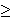1

Because we can either purchase some items or not purchase these items, each variable xi is 0 or 1 (called a binary variable). We can introduce similar constraints for each element in our universe (or on our grocery list). These inequalities (called constraints) have the form:

for each element e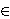U,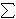i | eSi xi1

Our goal of minimizing the number of sets chosen (stores visited) can be stated by the objective function:
minimize1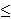in xi

So the mathematical formulation for this problem can be stated as

minimize1in xi
Subject to
for each element eU,i | eSi xi1
for each set i, xi{0, 1}.

Formulations of this type, where variables are restricted to a finite set (in this case the x variables being either 0 or 1) are called integer programs. Unfortunately, there is no easy way to solve these formulations either. However, there is a related problem which can be solved quickly.

Instead of restricting the x variables to the values of 0 or 1, we could allow them to take on any value within this range, i.e. 0xi1 for each set Si. Doing this converts the problem from an integer programming problem into a linear programming problem (called the LP-Relaxation), which can be solved quickly. The issue with this method though is that the solution obtained by an LP-Relaxation is not guaranteed to be an integer. In this case, how do we interpret the values xi?

Randomized Rounding Method

One approach to dealing with a non-integer solution to the LP-Relaxation is to treat the xi values as probabilities. We can say that xi is the probability that we select set i. This works because each value of xi is in the range of 0 to 1, which is necessary for a probability. We need to repeatedly select sets with their associated probabilities until all elements in our universe are covered. Selecting our sets based on this procedure is the randomized rounding approach.

Deterministic Rounding Method

A second approach to dealing with a non-integer solution to the LP-Relaxation is to base our solution on the most occurring element. If we let f be this frequency (i.e.the number of sets that the most occurring element occurs in), then we can define a solution by selecting set i if the LP=Relaxation solution gives the variable xi a value of at least (1/f).

None of these three approaches is guaranteed to give an optimal solution to an instance of this problem. I will not go into it in this post, but these can all be shown to be within some guaranteed range of the optimal solution, thus making them approximation algorithms.

Hope you enjoy.

# Learn About Binary Search Trees

These data structures are organized such that the data lies in “nodes” and each node connects directly to up to two new nodes. These new nodes are called the children of the node, and the original node is called the parent. Because there are up to two children, we designate one child as the “left” child, and the other as the “right” child with the properties that the value stored in the left child is less than the value in the parent, which in itself is less than the value of its right child. If a parent has less than two children, then one (or both) of its children are given the value of null.

The insert and delete procedures need to make sure that they keep the elements of a binary search tree in sorted order.
To insert into a BST, we must first find the correct location where the new element will be placed. This means comparing the value of the new element to the current head of the tree, resulting in three possible outcomes.
if the head is null, then insert the new node at the current position because there is no subtree to compare it to.
if the value of the new element is less than the value at the head node, run the insert procedure on the left child of head.
if the value of the new element is greater than the value at the head node, run the insert procedure on the right child of head.

Similarly, the remove procedure for a binary search tree must first find the element to be removed. Once that element is found, there are three cases depending on the type of node we are dealing with.
if the node has no children, then simply remove the node from the tree.
if the node has only one child (either a left child or a right child), then have the parent of the node point to the child of the node (thus bypassing the node itself).
if the node has two children, then we have two options, either replace the node with the minimum value of the right subtree or the maximum value of the left subtree. The nodes that have these minimum and maximum values will have at most one child because by definition a value less than the minimum value in a right subtree would be a left child and thus would be less than the minimum value, contradicting the meaning of a minimum value. Because these nodes have at most one child, we can now use the procedures above to remove these nodes from the tree.
Because a binary search tree is different than a standard array, there are different methods for viewing the its contents. Three common such methods are preorder, inorder, and postorder traversal.
Preorder traversal visits the nodes of a binary search tree in the order (node), (left child), (right child).
Inorder traversal visits the nodes of a binary search tree in the order (left child), (node), (right child).
Postorder traversal visits the nodes of a binary search tree in the order (left child), (right child), (node).

We are also interested in the depth of a tree, which amounts to the amount of layers or levels of the tree. This can be computed by counting the longest path from the root of the tree to a leaf node (a node with no children) in the tree.

Other Blogs that have covered this topic:
Stoimen’s Web Log

# Sorting Algorithms (Take Two)

Sorting is an essential part of our everyday lives. Because of this importance, many people have devoted much time towards improving the performance of different sorting procedures. Previously, I wrote a script comparing many of these algorithms. I decided to break that single script up into several scripts (one for each algorithm) so that the individual algorithms are easier to access and learn more about.

BubbleSort
When I first learned about BubbleSort, I thought of being in elementary school and how my teacher would arrange students in line by increasing height. The way she did this was to have us begin by standing in line (in arbitrary order). Then she would start at the beginning of the line and compare the height of each student to that of the student behind him. If the student in front was taller, then she’d have the two students change places. She continued doing this until she reached the back of the line, taking note of whether or not two students had moved or not. If a swap had been made, she would go back to the beginning of the line and start again.

What my teacher was doing (knowingly or unknowingly) was executing the BubbleSort algorithm. A more formal pseudocode version of this algorithm is listed below:

BubbleSort(A, start, end):
swapped <- false while (swapped is false) for (i = start to end) if (A[i] > A[i+1]) then
temp <- A[i] A[i] <- A[i+1] A[i+1] <- temp swapped <- true end if end for end while end BubbleSort Here is an execution of the BubbleSort algorithm.

SelectionSort
One of the more common ways that we do sorting in real life is through something similar to selection sort. Suppose we have a list of graded exams and want to have them in descending order before we pass them back to the students. The idea behind selection sort is to search for the largest element in the list, or in this case the highest test score, move that element to the beginning of the list, and then repeat the same procedure on the remaining portion of the list.

Here is a more formal pseudocode for the SelectionSort algorithm:

SelectionSort(A, start, end):
for (i = start to end)
min <- i for (j = i+1 to end) if (A[j] < A[min]) min <- j end if end for temp <- A[i] A[i] <- A[min] A[min] <- temp end for end SelectionSort Here is an execution of the SelectionSort algorithm

InsertionSort
If we think of the same set of (unsorted) graded exams as in the SelectionSort description that we wish to have them in descending order before we pass them back to the students, an alternative way to sort the exams is to have more of a “sort-as-you-go” type of a procedure where each test is only compared to an already sorted subset of the exams. The way this is carried out is to divide the exams into two piles. Originally, one pile (call it Pile 1) is empty and the other pile (Pile 2) contains the entire set of exams. We take the exam from the top of Pile 2 and compare it to the exams in Pile 1 and place it in the proper location in this pile (removing it from Pile 1). We continue this until Pile 2 is empty, in which case Pile 1 will have all the exams and the set of exams will be sorted.

Here is the pseudocode for the InsertionSort algorithm:
InsertionSort(A, start, end):
for (i = start to end)
val <- A[i] j <- i while (A[j - 1] > val)
A[j] <- A[j-1] j <- j - 1 end while A[j] <- val end for end InsertionSort Here is an execution of the InsertionSort algorithm

MergeSort
MergeSort is based on a simple “Divide and Conquer” approach. The principle behind it is simple: We want to sort an entire list, but if this entire list is sorted, then each half of this list must also be sorted. It is an easier problem to sort a smaller list than a larger list, so we sort each of the smaller lists. Once these two lists are sorted, we run a merge algorithm which combines the two smaller lists into one larger list. These smaller lists are then sorted using the same principle until our smaller lists are of size 1 or 2, in which case we can simply swap the two items or return the item itself.

The algorithm itself is similar to the hierarchy at work.
The boss is given a job – “sort these 8 documents in decreasing order”.
Boss divides the list into two sets of size 8 and gets two subordinates and says “hey you two, each of you take one of these lists and sort it and get it back to me”.
Each of subordinates then divide their lists into two distinct lists of size 4 and gets two sub-subordinates to sort these smaller lists.
The sub-subordinates then divide their lists into two distinct lists of size 2 and gets two sub-sub-subordinates to sort these smaller lists.
The sub-sub-subordinates now have lists of size 2 which are easy to sort, if they’re ordered, then they say “its already sorted”; if they’re not ordered, they swap the elements and say “here you go”.
Now that the lists of size 2 have been sorted, the sub-subordinates need to combine them into a list of size 4. This can be done by simply reading both lists and taking the maximum element from each list.
Similarly, the subordinates can just combine the lists of size 4 into lists of size 8 using that same principle.
Finally the boss can take the two lists of size 8 and combine it into a sorted list of size 16 using that same principle.

Here is the pseudocode for the MergeSort Algorithm:
MergeSort(A, start, end)
if ((end – start + 1) = 1)
return A
end if

mid <- (end - start) / 2 left <- {A[start], A[start+1], ... A[mid]} right <- {A[mid+1], A[mid+2], ... A[end] left <- MergeSort(left, 0, mid) right <- MergeSort(right, 0, end-mid) return Merge(left, right) end MergeSort Here is an execution of the MergeSort algorithm

QuickSort
QuickSort is considered the best sorting algorithm among the ones listed here. Like MergeSort, it operates under a divide and conquer principle. Unlike MergeSort, however, the question of where to divide the list (known as the pivot element) and the recursive calls are a bit more complex decisions.

The algorithm works by choosing a pivot element in the list (by default we can let this be the middle element in the list, but there are more complex variations that help decide what this should be), and then reordering the list so that the elements to the left (call this sub-list “small”) of the pivot element are all less than the pivot element, and the elements to the right (call this sub-list “big”) of the pivot element are all greater than the pivot element. So after the swap part of quicksort, the largest element of “small” will be less than the smallest element of “big”. We then need to check on whether the sub-lists “small” and “big” need to be sorted, which is true if we have not considered those regions yet. In such a case, we call the procedure Quicksort on each of the sub-lists.

Here is the pseudocode for the QuickSort Algorithm:
QuickSort(A, left, right)
i <- left j <- right pivot <- A[floor((left + right) / 2)] while (i <= j) while (A[i] < pivot) i <- i+1 end while while (A[j] > pivot)
j <- j-1 end while if (i <= j) tmp <- A[i] A[i] <- A[j] A[j] <- tmp i <- i+1 j <- j-1 end if end while if (left < j) A <- QuickSort(A, left, j) end if if (i < right) A <- QuickSort(A, i, right) end if return A; end QuickSort Here is an execution of the QuickSort algorithm

# Sorting Algorithms

I just added a script which executes and gives examples of some basic sorting algorithms. Its accessible at Sorting Algorithms

Right now, the algorithms consist of BubbleSort, InsertionSort, MergeSort and SelectionSort.

BubbleSort works by comparing items that are next to one another. If the items are not in proper order, they are swapped. The process continues until we pass through the list without making a swap.

InsertionSort divides an array into two parts, a sorted part and an unsorted part. In each iteration, a new element is compared to all the elements of the sorted part of the array to find where it belongs in this subarray. The algorithm terminates when all elements have been inserted into the sorted part of the array.

MergeSort is based on the divide and conquer algorithm. It works by calling itself (the function mergesort) on two smaller arrays: the elements in the first half of the array, and the elements in the second half of the array.

QuickSort is another divide and conquer algorithm. It is based on first choosing a pivot element (we choose the middle element, but it can be any element) and ensuring that all elements that are less than the pivot element are to the left of it in the array, and all elements greater than the pivot element are to the right in the array. Then the quicksort algorithm is recursively called on each part of the array.

SelectionSort repeatedly finds the minimal value in the list and places it in the first (remaining) position in the (unsorted) array.

http://www.learninglover.com/examples.php?id=9